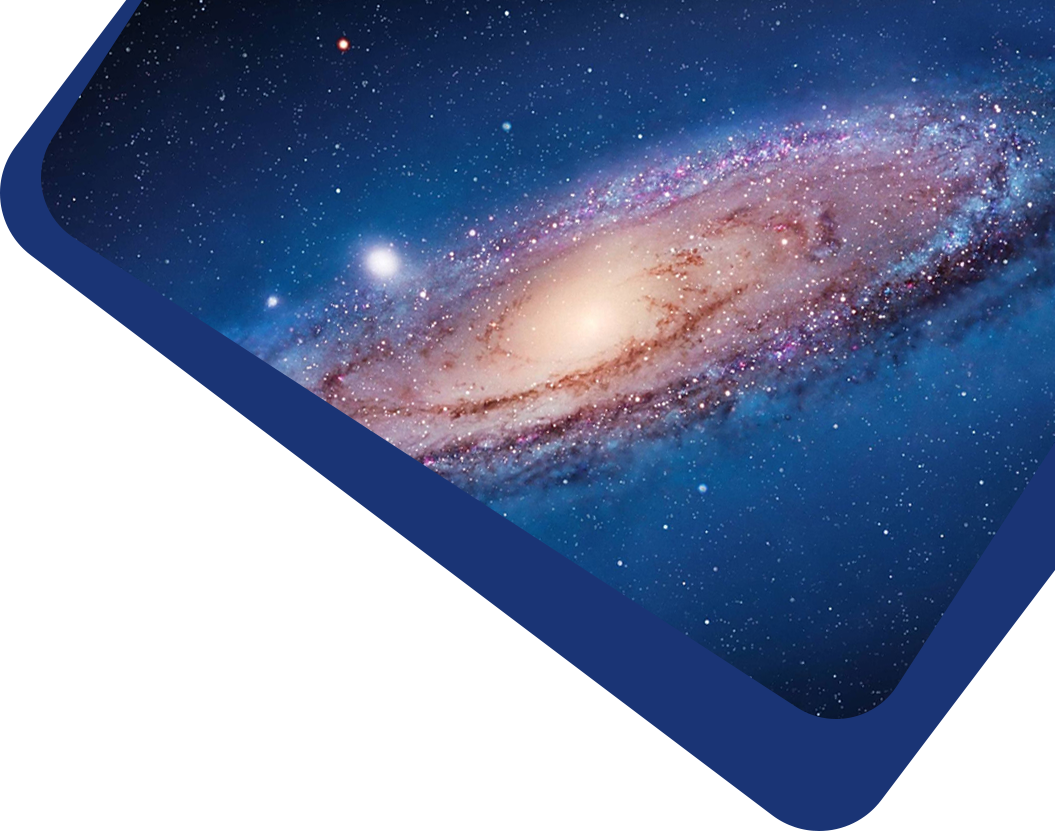Vol 22, No 5

## Constraining Brans–Dicke Cosmology with the CSST Galaxy Clustering Spectroscopic Survey

#### Anda Chen, Yan Gong, Fengquan Wu, Yougang Wang, Xuelei Chen

Abstract

Abstract The Brans–Dicke (BD) theory is the simplest Scalar-Tensor theory of gravity, which can be considered as a candidate of modified Einstein’s theory of general relativity. In this work, we forecast the constraints on BD theory in the CSST galaxy clustering spectroscopic survey with a magnitude limit ∼23 AB mag for point-source 5σ detection. We generate mock data based on the zCOSMOS catalog and consider the observational and instrumental effects of the CSST spectroscopic survey. We predict galaxy power spectra in the BD theory from z = 0 to 1.5, and the galaxy bias and other systematical parameters are also included. The Markov Chain Monte Carlo technique is employed to find the best-fits and probability distributions of the cosmological and systematical parameters. A BD parameter ζ is introduced, which satisfies $$\zeta=\ln\left(1+\frac{1}{\omega}\right)$$. We find that the CSST spectroscopic galaxy clustering survey can give |ζ| < 10−2 , or equivalently $$|\omega| > \mathcal{O}(10^2)$$ and $$|\dot{G}/G|<10^{-13}$$, under the assumption ζ = 0. These constraints are almost at the same order of magnitude compared to the joint constraints using the current cosmic microwave background, baryon acoustic oscillations and Type Ia supernova data, indicating that the CSST galaxy clustering spectroscopic survey would be powerful for constraining the BD theory and other modified gravity theories.

Keywords

Keywords cosmology – cosmological models – cosmological parameters from large-scale structure

Full Text
Refbacks

• There are currently no refbacks.